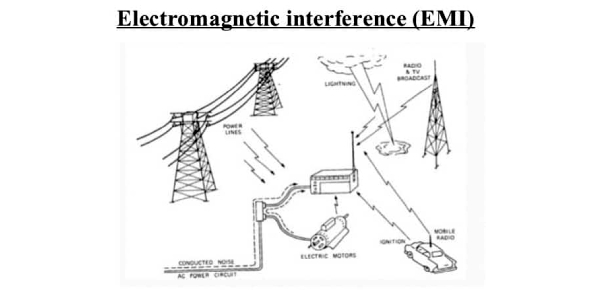# Engineering Quiz: Electromagnetic Interference (EMI)

8 Questions | Total Attempts: 5341SettingsMost of the things today are being controlled by electricity, and one of the ways through which this equipment may stop functioning is through the introduction of electromagnetic interference. EMI is any frequency of electrical noise. What do you know about electromagnetic interference (EMI)? Take up the quiz below and get to learn more about it. All the best!

• 1.
You will find all the background information needed here.
• 2.
Scientists concluded from another experiment that fiberglass was the best insulator because it was the poorest heat conductor. Does the data from this experiment support their conclusion?
• A.

No, because asbestos conducted less heat than fiberglass

• B.

Yes, because the heat flow for fiberglass was negative in some cases

• C.

Yes, because the fiberglass had the highest initial temperature in trial 2

• D.

Yes, because the fiberglass insulator was tested multiple times

• 3.
The scientists in this experiment hypothesized that thicker insulators would conduct less heat. Did the data in this experiment support their conclusion?
• A.

Yes, because for each material, the thicker insulator had less heat flow

• B.

Yes, because the final temperature was always higher with thick versus thin insulator

• C.

No, because the heat flow depended only on the type of material, not thickness

• D.

No, because the heat flow depended only on the temperature of the oven

• 4.
A student hypothesizes that when the outside temperature is lower than the starting temperature of the water, then the heat will travel out of the water into the surroundings. Does the data support this hypothesis?
• A.

Yes, because the heat flow is negative when the oven temperature is lower than the initial temperature of the water

• B.

Yes, because the heat flow is positive when the oven temperature is lower than the initial temperature of the water

• C.

No, because the heat flow depends only on the type and thickness of material used as an insulator

• D.

No, because the heat flow is negative when the oven temperature is higher than the initial temperature of the water

• 5.
A student concluded that the worst insulator tested was rubber, because it had the highest heat conductance. Does the data from this experiment support this conclusion?
• A.

Yes, because the heat flow for rubber was greater than any other material

• B.

Yes, because the heat flow for rubber was less than any other material

• C.

No, because the heat flow for rubber was the greatest of any material

• D.

No, because the asbestos insulators had the lowest heat flow

• 6.
The output power of the cascaded amplifier/attenuator system can be determined using
• A.

Actual gain of amplifier

• B.

Actual gain of amplifier and attenuator

• C.

Gain in dB of amplifier and attenuator

• D.

Actual gain of attenuator

• 7.
LISN is a device used to measure conducted emissions. LISN stands for
• A.

Line integrated stabilization network

• B.

Line impedance stabilization network

• C.

Line integrated stored network

• D.

Laser integrated stabilization networking

• 8.
In EMC signal, the source delivers maximum power to the input of the transmission line when the transmission line input impedance
• A.

Is equal to the source resistance

• B.

Greater than the source resistance

• C.

Smaller than the source resistance

• D.

None of these

Related TopicsBack to top